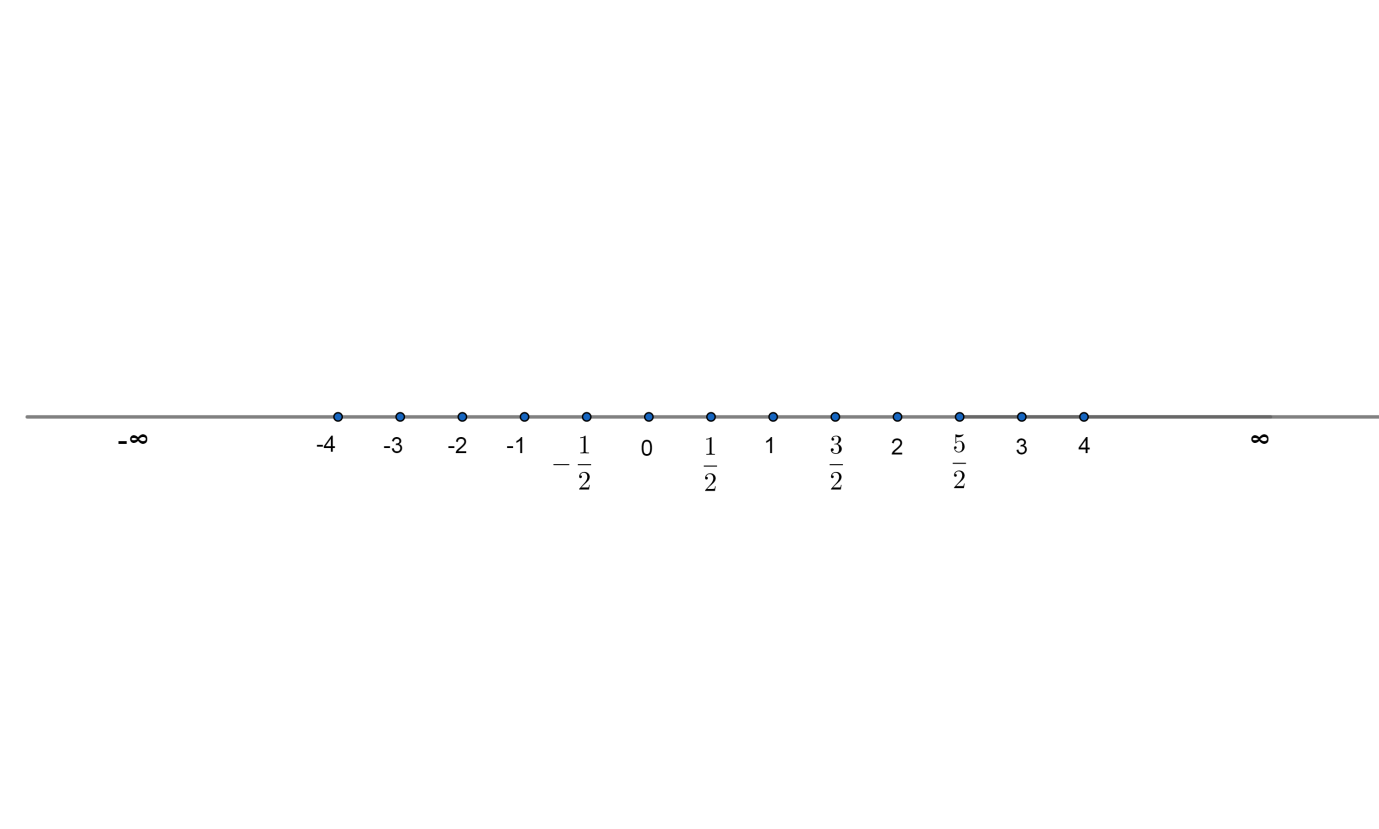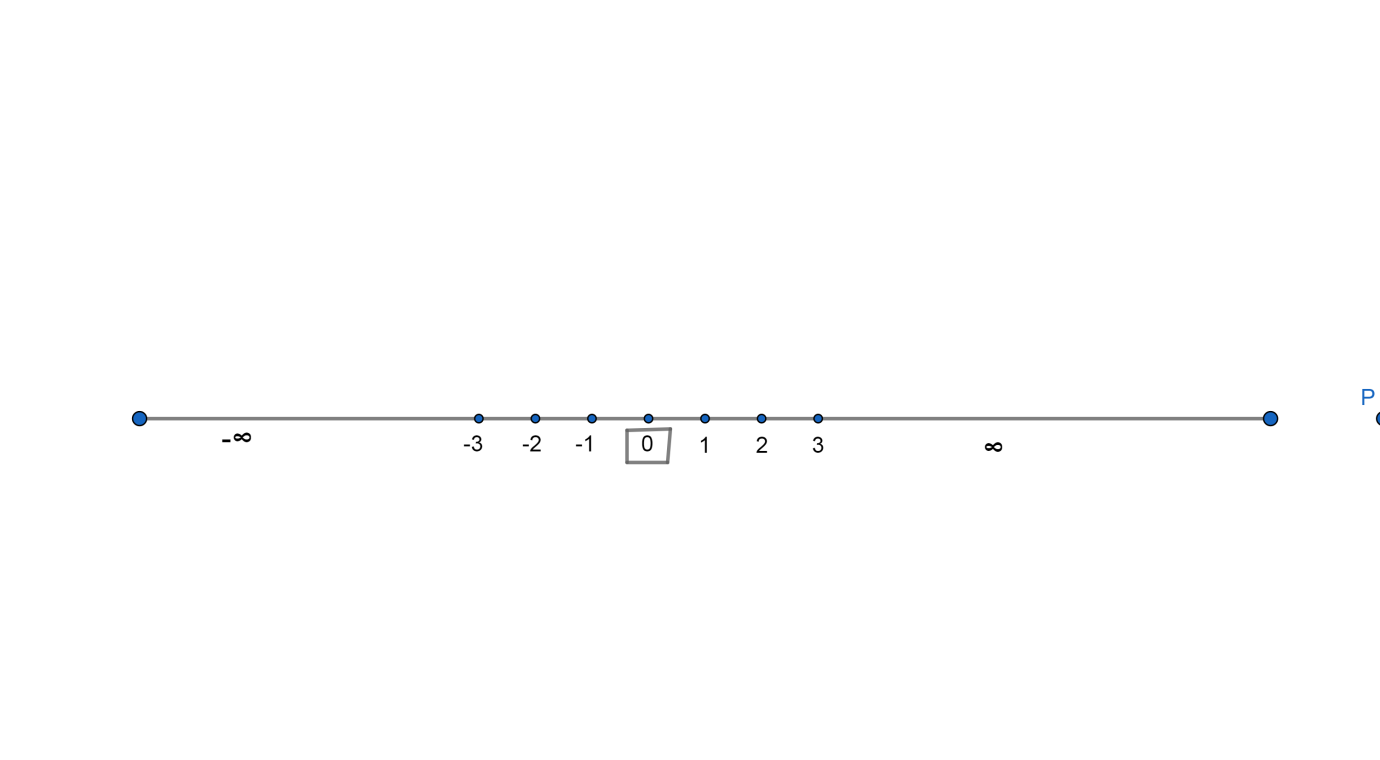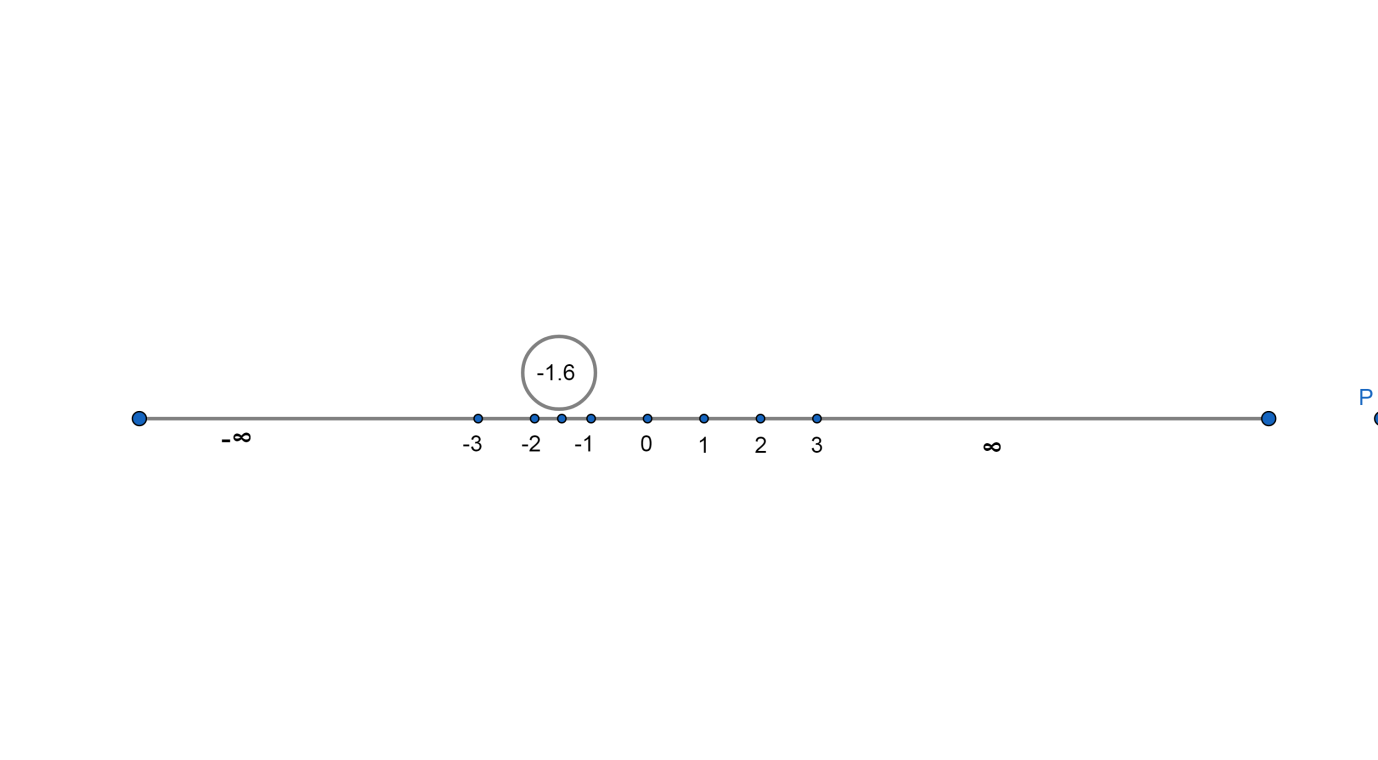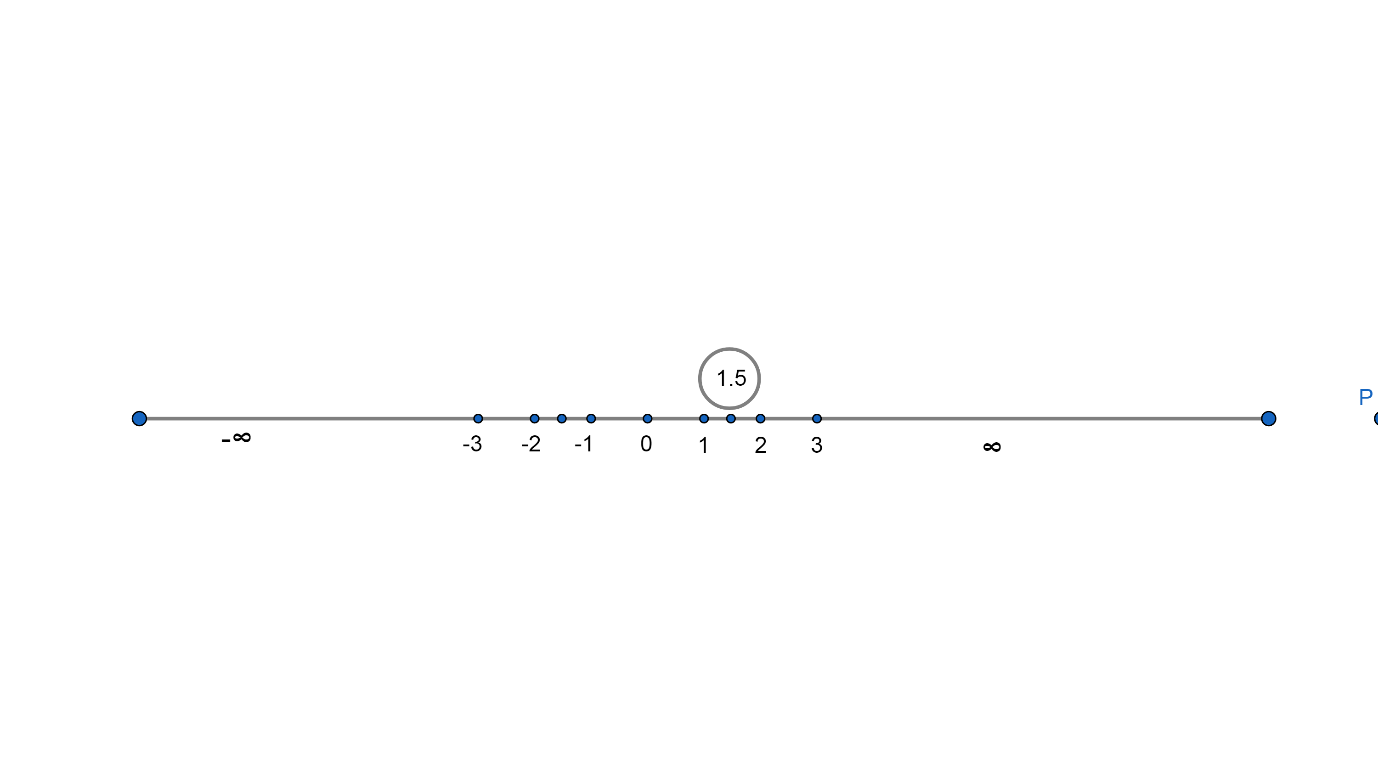Courses
Courses for Kids
Free study material
Free LIVE classes
MoreLIVE
Join Vedantu’s FREE Mastercalss

# Find a rational number between -1 and 1.A. 0B. $\dfrac{1}{\sqrt{-2}}$ C. $\dfrac{-8}{5}$D. $\dfrac{3}{2}$Verified
359.1k+ views
Hint: First of all check if a number is rational as not by checking that it should be of the form $\dfrac{p}{q}$ where p and q are integers and $q\ne 0$.

Then check if that number is between -1 and 1 in the number line.
Here we have to find the rational number between -1 to 1 out of given options.
Before proceeding with the question we must know what a rational number is.
A rational number is a number that can be expressed in the form of $\dfrac{p}{q}$ where p and q are integers and $q\ne 0$. Since q may be equal to 1, hence every integer is a rational number. Examples are $0,1,2,\dfrac{2}{3},-4,\dfrac{-6}{7},etc$
Now we will see what a number line is. A number line is a straight line with numbers placed at equal intervals or segments along its length. A number line contains all real numbers that are rational numbers and irrational numbers both.
We can show number line asNow we will find the rational number between -1 and 1 out of given options.
(A) 0

Since we know that all integers are rational numbers. Therefore, 0 is also a rational number.
Also we know that 0 lies between -1 and 1 in number line as shown,Therefore, we can say that 0 is a rational number between -1 and 1. Therefore, this option is correct.
(B) $\dfrac{1}{\sqrt{-2}}$
Since we know that in any rational number of form $\dfrac{p}{q}$, p and q must be integer but here $\sqrt{-2}$ is not an integer but an imaginary number, so $\dfrac{1}{\sqrt{-2}}$is not a rational number. Therefore, this option is incorrect.
(C) $\dfrac{-8}{5}$
$\dfrac{-8}{5}$is a rational number because it is in form of $\dfrac{p}{q}$that is $\dfrac{-8}{5}$where -8 and 5 are integers.
In decimal form we can write $\dfrac{-8}{5}=-1.6$
In number line we can show – 1.6 or $\dfrac{-8}{5}$ asSince – 1.6 or $\dfrac{-8}{5}$ does not lie between – 1 and 1. So this option is incorrect.
(D) $\dfrac{3}{2}$
$\dfrac{3}{2}$is a rational number because it is in form of $\dfrac{p}{q}$ that is $\dfrac{3}{2}$where 3 and 2 are integers.

In decimal form we can write $\dfrac{3}{2}=1.5$
In number line, we can show 1.5 or $\dfrac{3}{2}$ as;Since, 1.5 or $\dfrac{3}{2}$does not lie between -1 and 1.
So this option is incorrect.
Hence option (A) is correct.

Note: Students must note that there are infinitely many rational numbers between any two numbers but here we must check from option to get rational numbers -1 and 1. Before checking if a number lies between -1 and 1 or not, students must check if it is rational or not. It is always better to convert fractional form to decimal form to judge the magnitude of a number correctly.
Last updated date: 21st Sep 2023
Total views: 359.1k
Views today: 7.59k